• 看了这一篇知乎文章，又加深了一遍印象 https://www.zhihu.com/question/27126057
看了这一篇知乎文章，又加深了一遍印象
https://www.zhihu.com/question/27126057
为什么不用平方损失函数，原因是使用平方损失函数，会存在很多局部极小点，梯度下降很容易陷入局部最优
https://www.zhihu.com/question/264396874/answer/281443787
展开全文逻辑回归
• 【学习任务】 ...Logistic回归为什么不像线性回归那样用平方损失函数？ Logistic回归的参数为什么不像线性回归那样直接公式求解？ Logistic回归与线性回归有哪些联系？ 一.Logisti...


Logistic回归损失函数推导
前言Logistic回归损失函数的极大似然推导：西瓜书公式3.27怎么推来的？Logistic回归损失函数的极大似然推导：西瓜书公式3.27怎么推来的？Logistic回归损失函数的最优化算法：什么是牛顿法、拟牛顿法？为什么不用线性回归做分类？Logistic回归为什么不像线性回归那样用平方损失函数？Logistic回归的参数为什么不像线性回归那样直接公式求解？Logistic回归与线性回归有哪些联系？

前言
在Logistic回归的极大似然推导之前，我们先要明白，什么是Logistic回归？ 回归问题可以分成如下三类：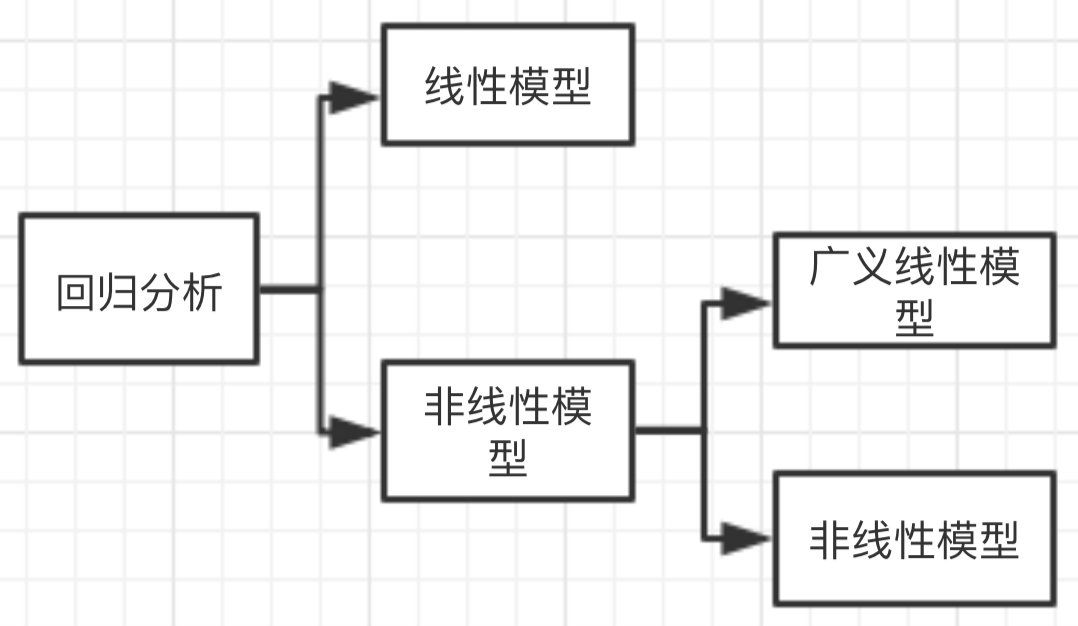其中广义线性回归，本质上是非线性回归，但我们可以把它转变为线性回归来做。而Logistic回归就是广义线性回归的一种。那为什么广义线性回归可以将非线性转变为线性来做呢？
广义线性回归实质上是线性回归的扩展，其要求因变量只能通过线性的形式来依赖于自变量，从而保持了线性自变量的思想。那具体怎么以线性的形式依赖？广义线性通过设定一个连接函数，将因变量的期望与因变量相联系，并且对误差的分布给出了一个误差函数。
因此对于广义线性回归模型而言，其有三部分构成： 第一是线性自变量，描述了自变量与因变量的直接关系； 第二是连接函数，描述了因变量与因变量的期望之间的关系； 第三是误差函数，描述了广义线性模型中的随机误差。
Logistic回归损失函数的极大似然推导：西瓜书公式3.27怎么推来的？
在说明清楚Logistic回归之前，我们先来讲清楚Logistic回归的广义，即对数线性回归(log-linear regression)是什么。 根据上节的内容，线性回归 我们知道线性回归表达的是真实标记y与自变量x的一一映射，那么，我们如何得到自变量x与y的期望值的一个映射呢？ 假设y的期望值是一个对数函数，那么我们可以知道：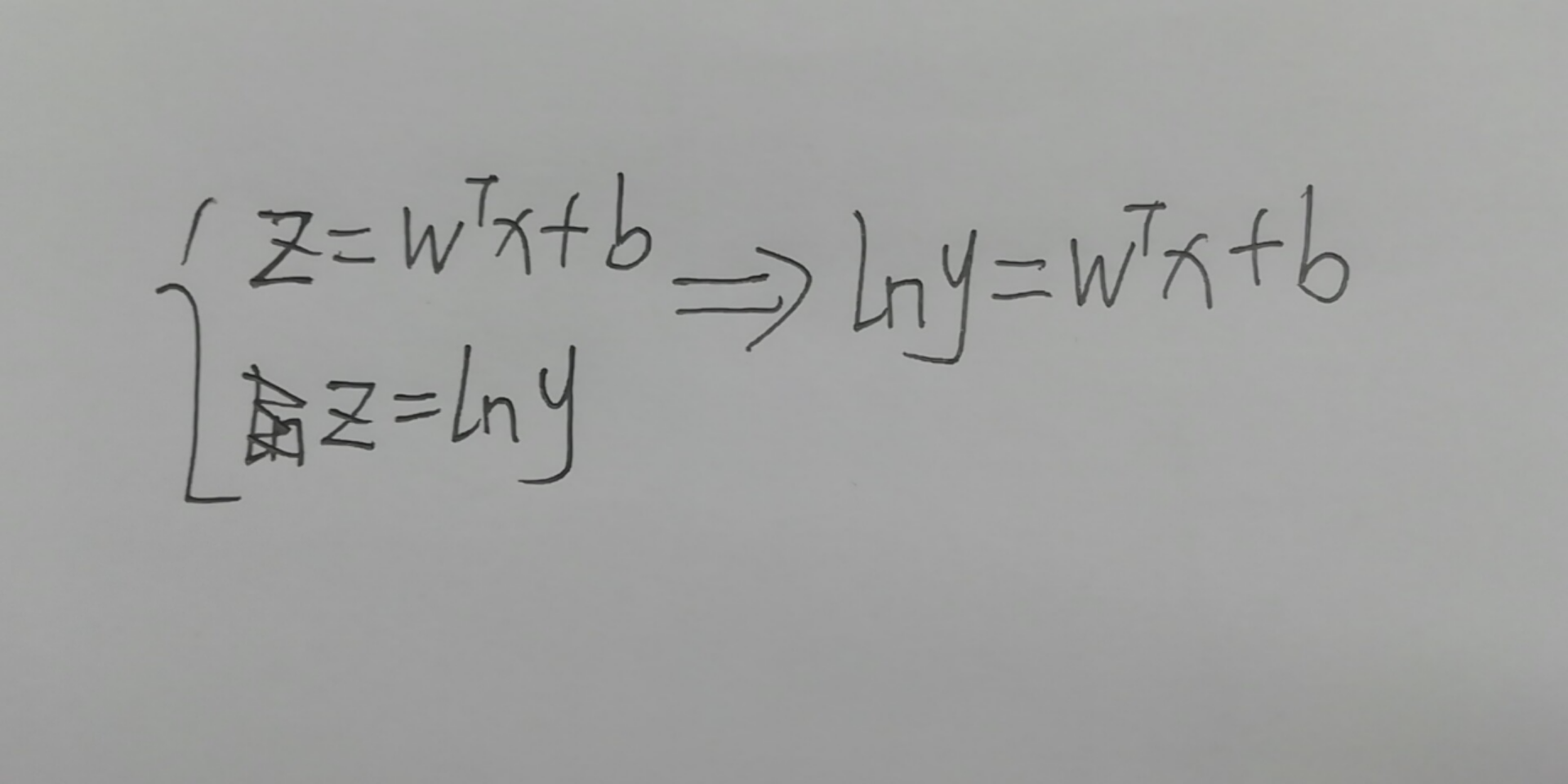于是得到了对数线性回归模型(log-linear regression),这里y的期望函数，即对数函数起到了将线性回归模型的预测值和真实标记联系起来的作用。
理解到这，再往下看对数几率回归(Logistic regression),即Logistic回归损失函数，其只是将y的期望函数，即连接函数改变为了sigmoid函数，那么连接函数的选择有没有什么要求呢？西瓜书上p57页提到，说对于“只需要找一个单调可微函数将分类任务的真实标记y与线性回归模型的预测值联系起来”。这里再多解释下，为什么要用单调可微？因为后期会需要求导，单调可微是为了让求导的局限性更小。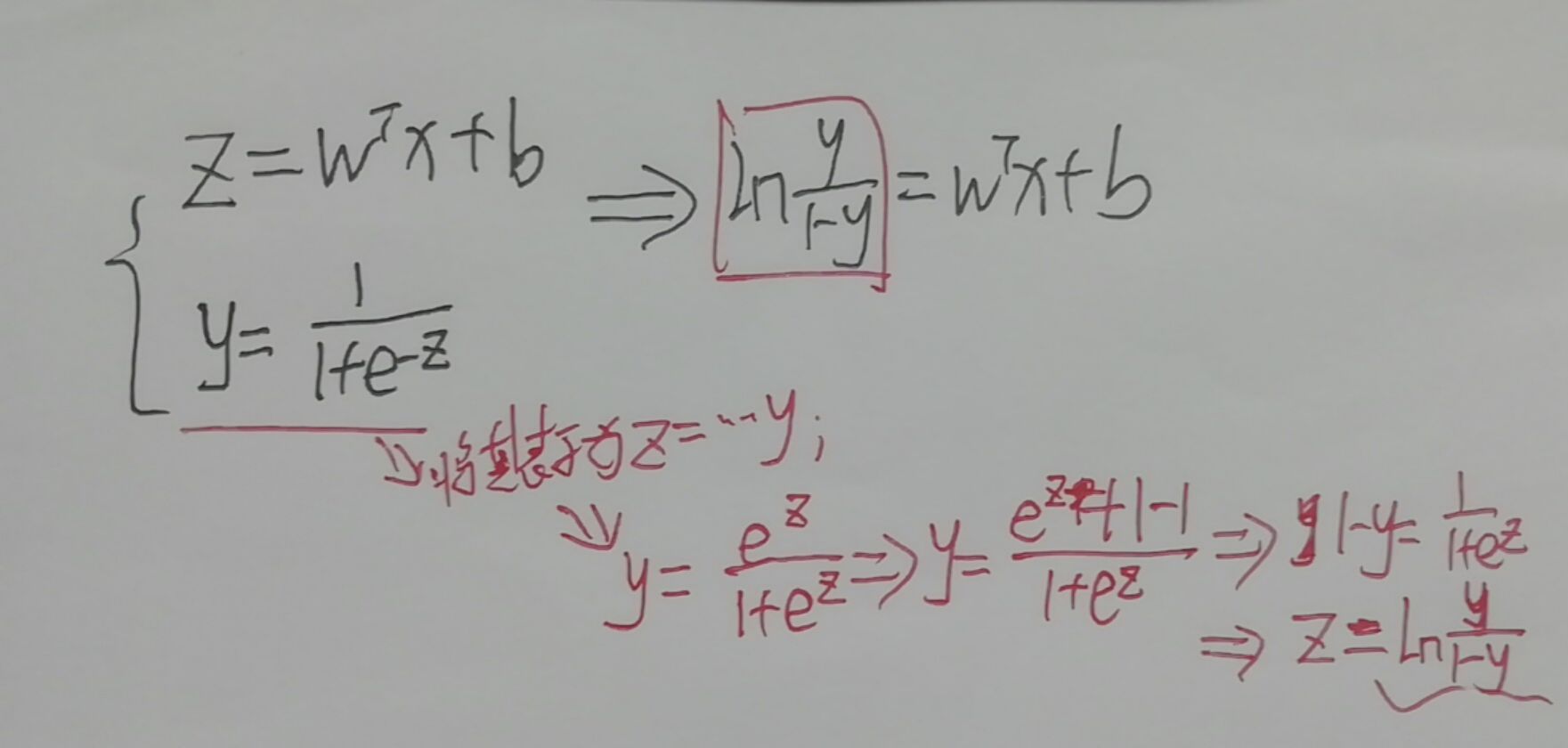到这里以后，我们仅仅是说出了Logistic回归是什么，但是它和极大似然又有什么毛关系呢？又为何用极大似然来求它的损失函数？ 我们先来看下sigmoid函数的特性：sigmoid函数对应的取值范围是0-1，即采用概率的方式来进行判断，正是基于此，因此在求概率的分布情况时，同上一篇博客一样，我们才可以通过极大似然估计来推导其损失函数，推导过程如下：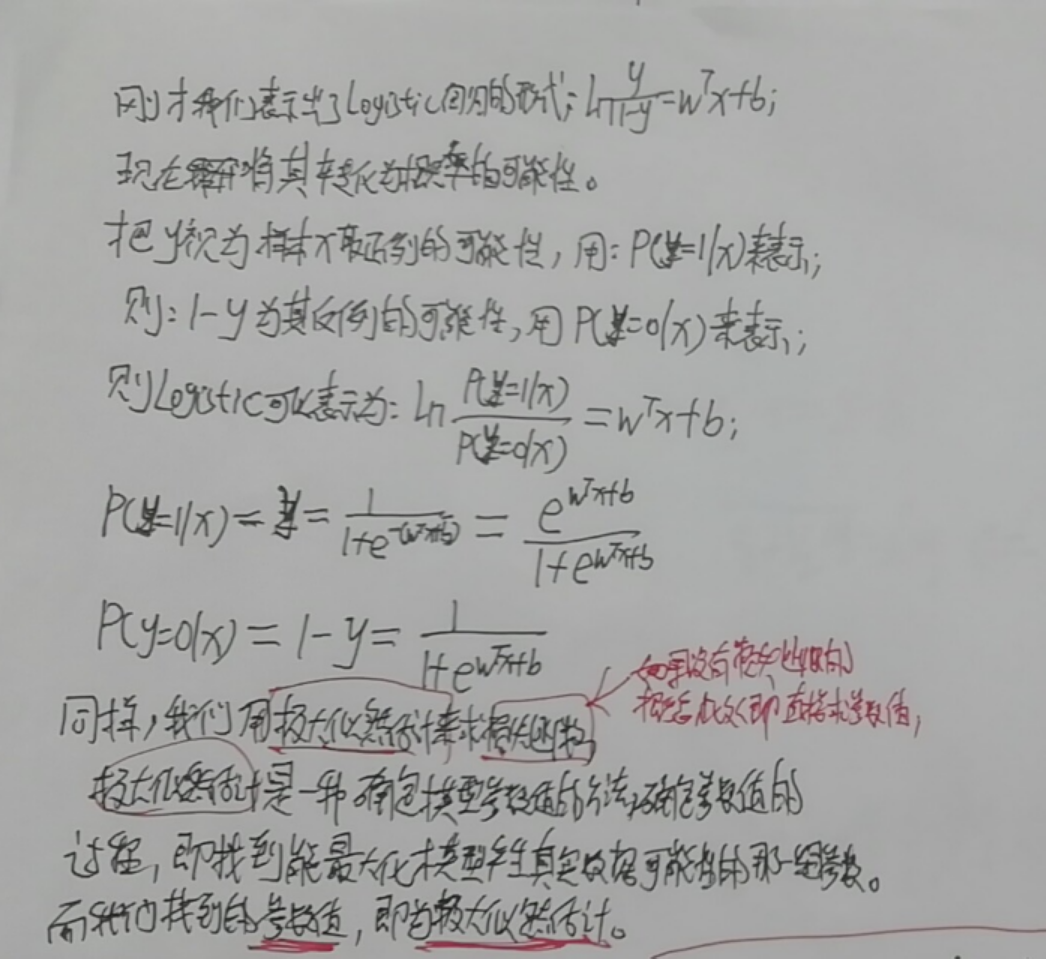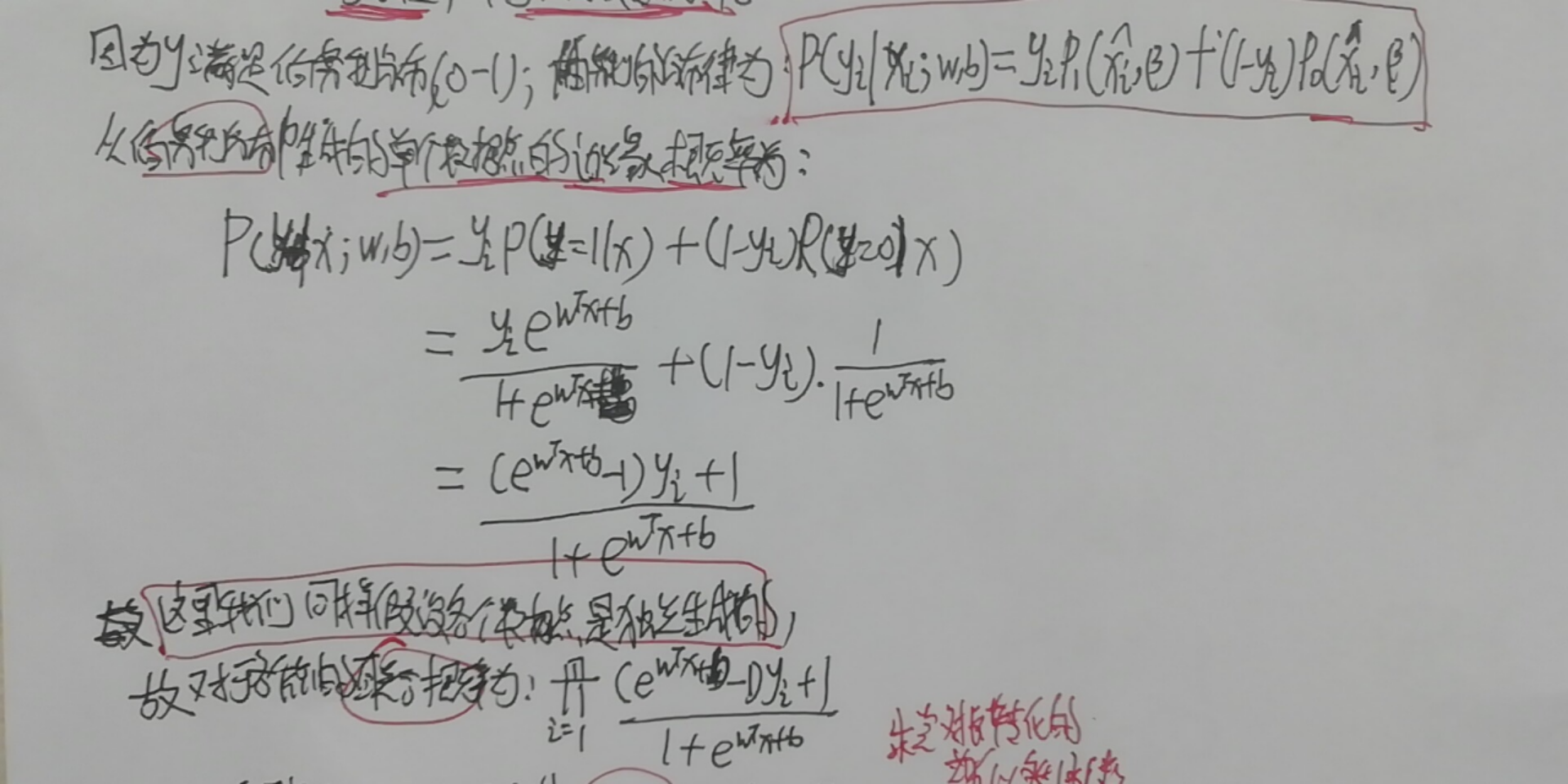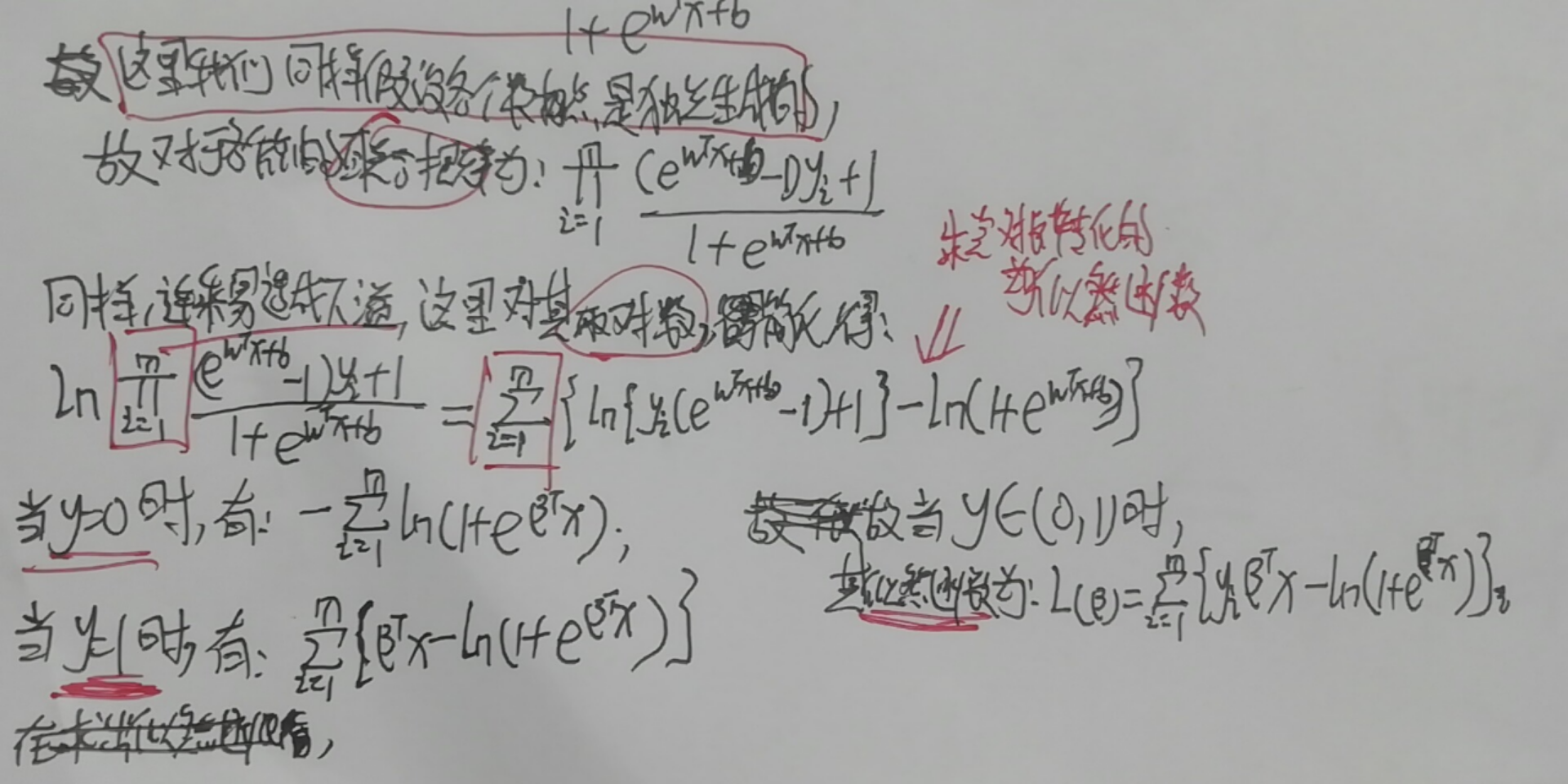在求出似然函数后，接下来要考虑的就是如何最大化似然函数以求出损失函数，因为根据最优化原理，任何最大化问题统一转为最小，任何凹函数都转为凸函数。所以将最大化似然函数变为最小化其相反的函数，并将其相反的函数作为损失函数，如下：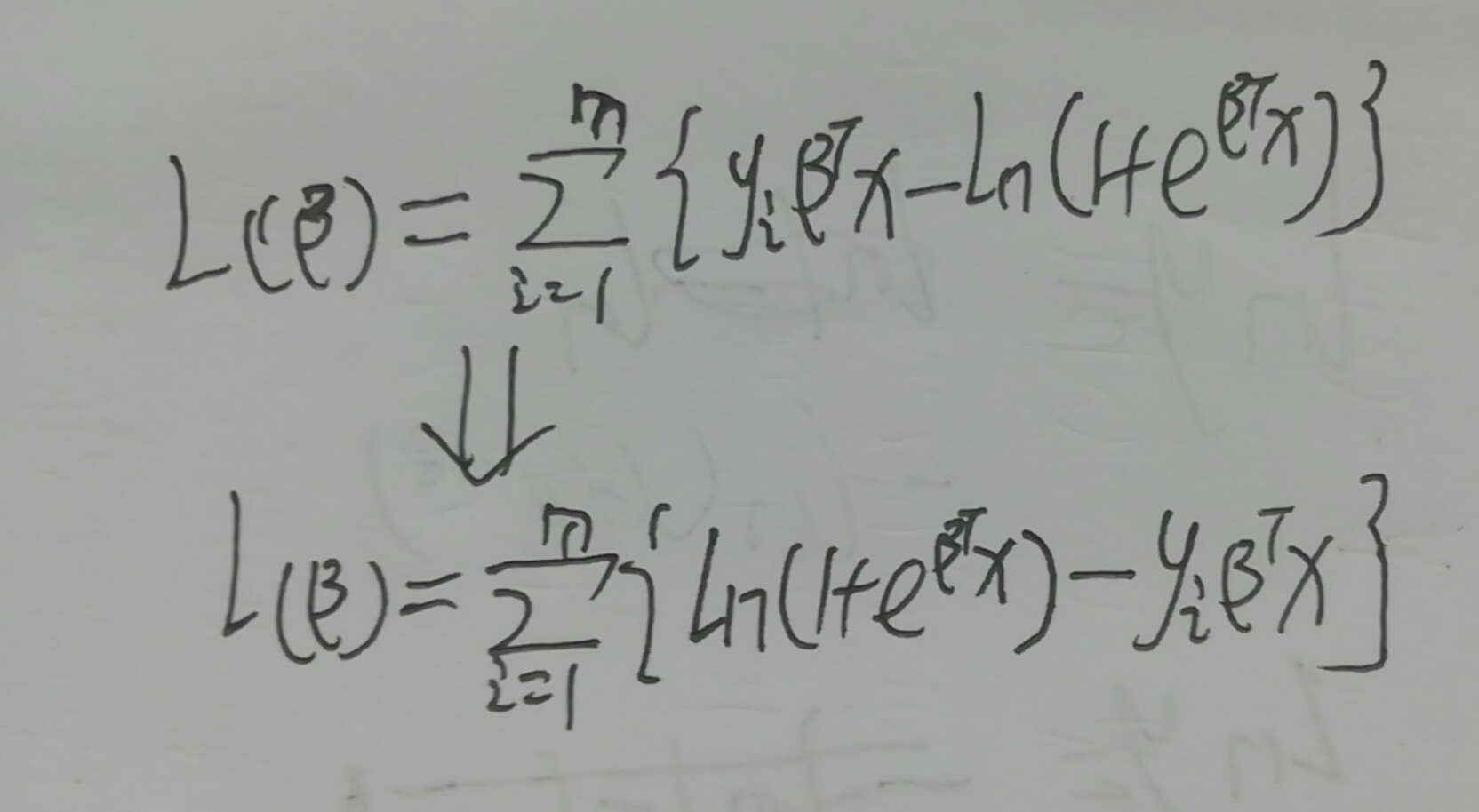对照西瓜书求解如下：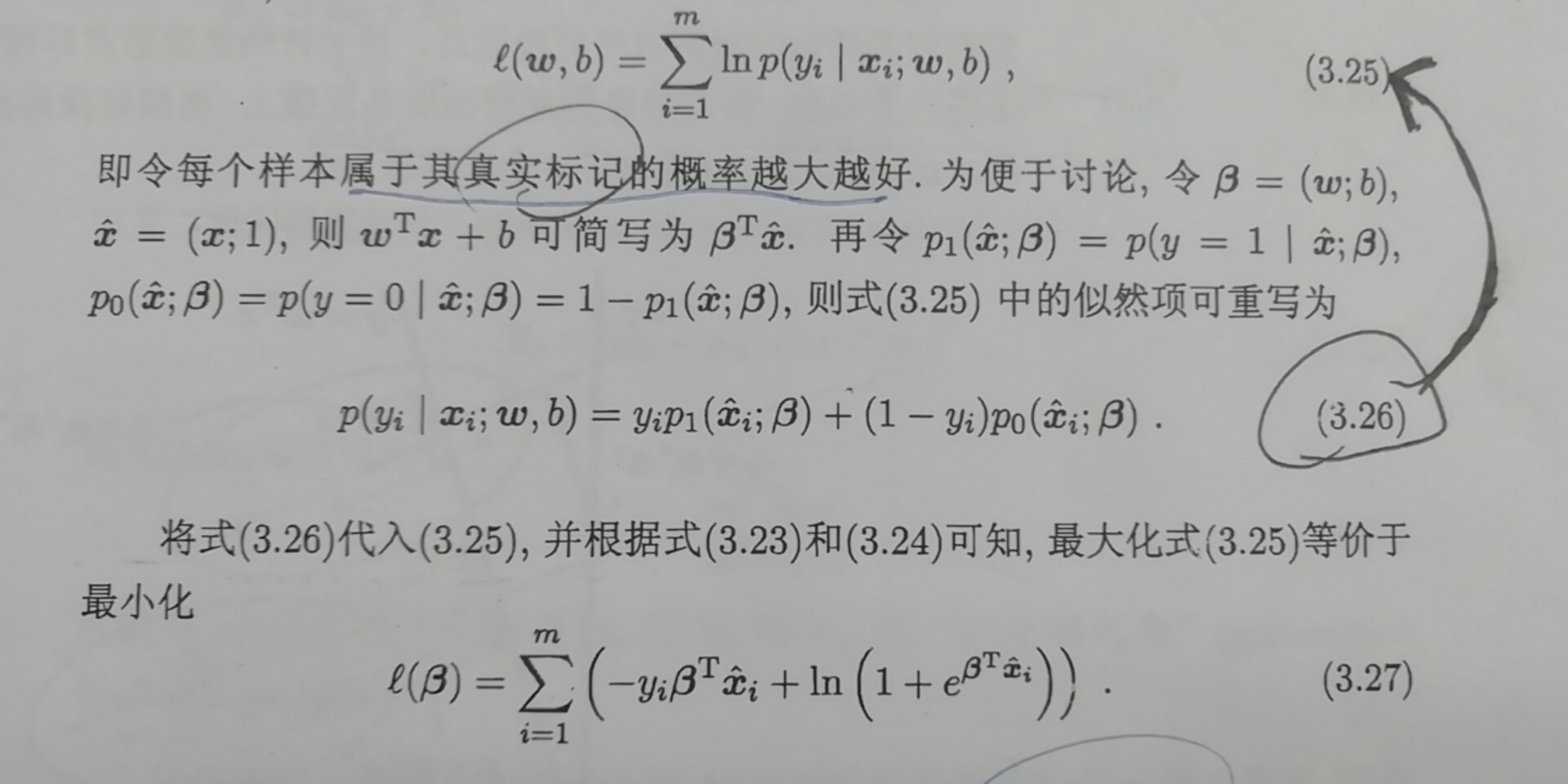总结->Logistic回归：就是用对数几率函数，将模型预测值z转化为接近0或1的y值，再用线性模型拟合y的对数几率。
Logistic回归损失函数的极大似然推导：西瓜书公式3.27怎么推来的？
Logistic回归损失函数的最优化算法：什么是牛顿法、拟牛顿法？
为什么不用线性回归做分类？
Logistic回归为什么不像线性回归那样用平方损失函数？
Logistic回归的参数为什么不像线性回归那样直接公式求解？
Logistic回归与线性回归有哪些联系？
展开全文Logistic回归
• 讲解平方损失函数与交叉熵损失函数 适用的情况以及 解释了原因。 特别是回归问题为什么不能使用 交叉熵损失函数。 博客链接：https://blog.csdn.net/m_buddy/article/details/80224409 平方损失函数与...
讲解平方损失函数与交叉熵损失函数 适用的情况以及 解释了原因。
特别是回归问题为什么不能使用 交叉熵损失函数。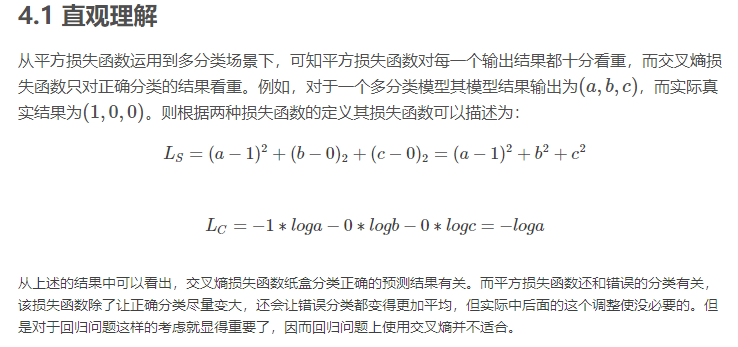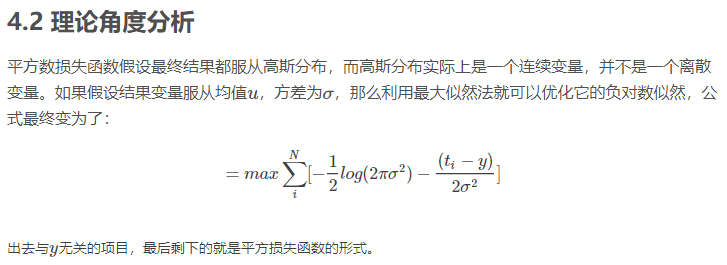博客链接：https://blog.csdn.net/m_buddy/article/details/80224409

平方损失函数与交叉熵损失函数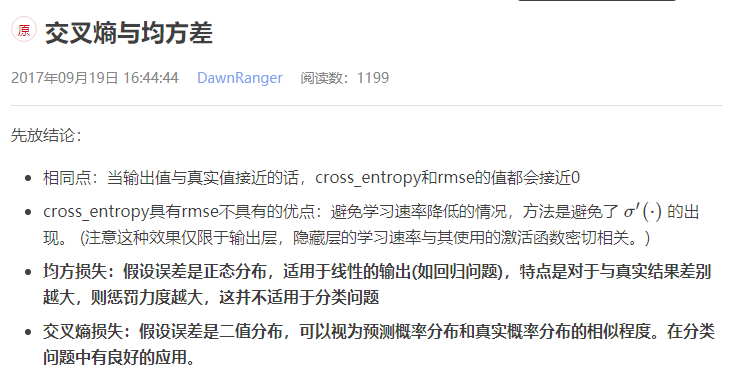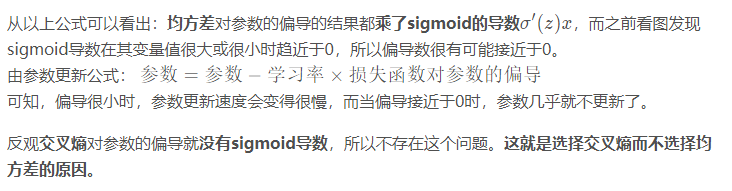交叉熵与均方差   https://blog.csdn.net/dawnranger/article/details/78031793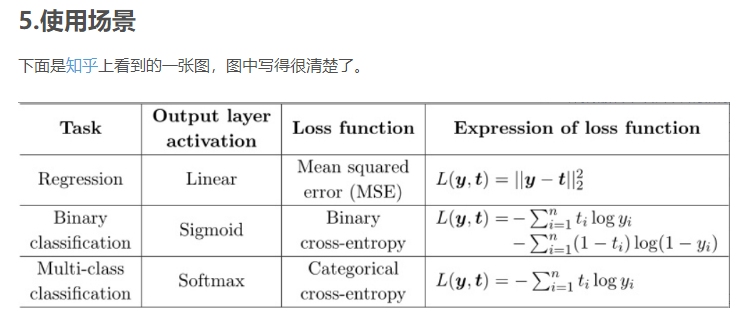这里的categorical cross-entropy其实就是 log 似然损失函数。
机器学习中的损失函数  https://blog.csdn.net/rosenor1/article/details/52302217
为什么交叉熵可以作为损失函数？  https://blog.csdn.net/iloveyousunna/article/details/79205347
softmax和sigmoid以及SVM   https://blog.csdn.net/qq_35550465/article/details/82704189
对数损失函数是如何度量损失的？  https://www.zhihu.com/question/27126057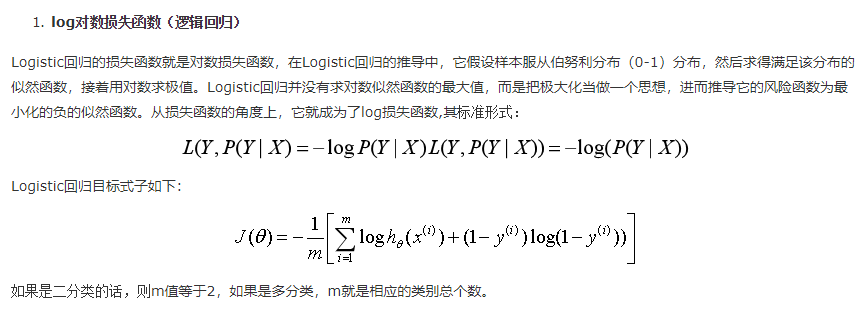机器学习中常见的损失函数   https://www.cnblogs.com/houjun/p/8956384.html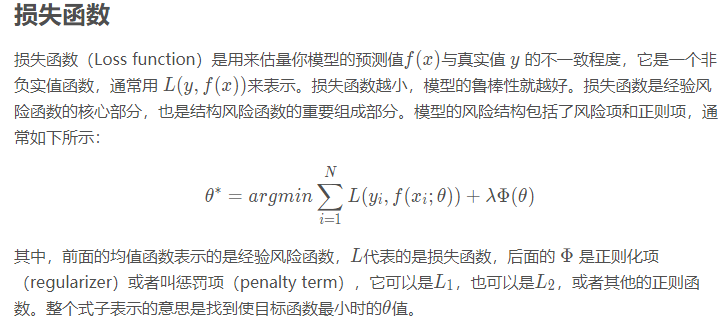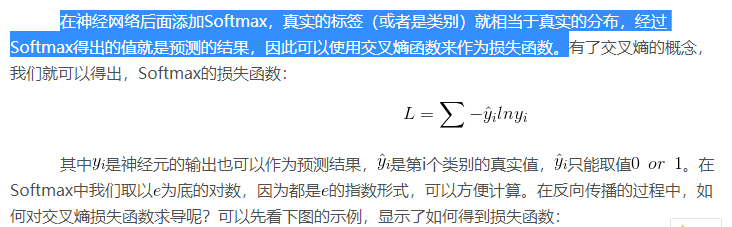以上图片来自： https://blog.csdn.net/hearthougan/article/details/82706834
softmax 交叉熵损失函数求导： https://www.jianshu.com/p/6e405cecd609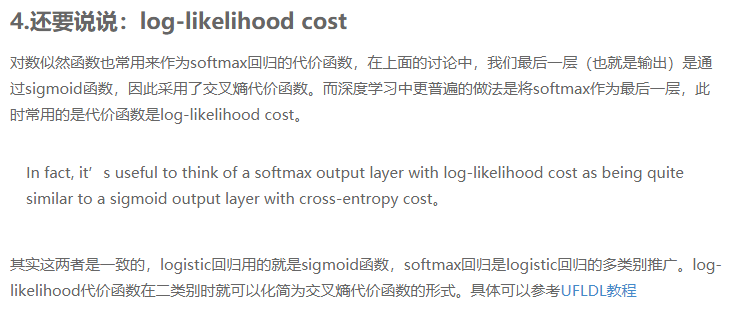https://blog.csdn.net/qingyang666/article/details/70245855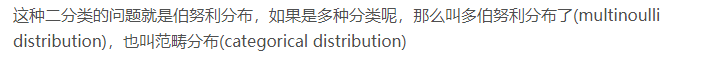https://www.cnblogs.com/klchang/p/9217551.html   对数损失函数(Logarithmic Loss Function)的原理和 Python 实现
用于Multinoulli（多项分布）输出分布的softmax单元   https://blog.csdn.net/weixin_40516558/article/details/79962674
多项式分布及Softmax回归模型推导   https://blog.csdn.net/liuyhoo/article/details/81542100

展开全文• 之前是根据函数图像推导损失函数为误差平方和，这次用统计学方法推导。 拟合数据，就是把误差减到最小 误差ϵ=y−θTXϵ=y−θTX\epsilon=y-\theta^TX。 假设误差服从正态分布，误差最小也就是期望为0。ϵϵ\...
把统计看了一遍就是为了这里!  线性回归假设函数为

y=θTX

y

=

θ

T

X

$y=\theta^TX$  之前是根据函数图像推导出损失函数为误差平方和，这次用统计学方法推导。  拟合数据，就是把误差减到最小  误差

ϵ=y−θTX

ϵ

=

y

−

θ

T

X

$\epsilon=y-\theta^TX$。  假设误差服从正态分布，误差最小也就是期望为0。

ϵ

ϵ

$\epsilon$~N(0,

σ2

σ

2

$\sigma^2$)  最大似然估计就是使所有样本最接近参数,也就是似然函数最大。  求似然函数

L(θ)=∏i=1mp(ϵ)=∏i=1m12π√σe−ϵ22σ2

L

(

θ

)

=

∏

i

=

1

m

p

(

ϵ

)

=

∏

i

=

1

m

1

2

π

σ

e

−

ϵ

2

2

σ

2

$L(\theta)=\prod\limits_{i=1}^{m}p(\epsilon)=\prod\limits_{i=1}^{m}{1\over{\sqrt{2\pi}\sigma}}e^{-{{\epsilon^2}\over{2\sigma^2}}}$

L(θ)=12π√m1σme−∑i=1mϵ22σ2

L

(

θ

)

=

1

2

π

m

1

σ

m

e

−

∑

i

=

1

m

ϵ

2

2

σ

2

$L(\theta)={1\over{\sqrt{2\pi}^m}}{1\over{\sigma}^m}e^{-{\sum\limits_{i=1}^{m}{\epsilon^2\over{2\sigma^2}}}}$

L(θ)=2π‾‾‾√−mσ−me−∑i=1m(y−θTX)22σ2

L

(

θ

)

=

2

π

−

m

σ

−

m

e

−

∑

i

=

1

m

(

y

−

θ

T

X

)

2

2

σ

2

$L(\theta)={{\sqrt{2\pi}^{-m}}}{{\sigma}^{-m}}e^{-{\sum\limits_{i=1}^{m}{(y-\theta^TX)^2\over{2\sigma^2}}}}$  两边取ln

lnL(θ)=−mln(2π‾‾‾√)−mln(σ)−12σ2∑i=1m(y−θTX)2

ln

⁡

L

(

θ

)

=

−

m

ln

⁡

(

2

π

)

−

m

l

n

(

σ

)

−

1

2

σ

2

∑

i

=

1

m

(

y

−

θ

T

X

)

2

$\ln L(\theta)=-m\ln(\sqrt{2\pi})-mln(\sigma)-{1\over{2\sigma^2}}{\sum\limits_{i=1}^{m}{(y-\theta^TX)^2}}$  要使似然函数最大，

∑i=1m(y−θTX)2

∑

i

=

1

m

(

y

−

θ

T

X

)

2

${\sum\limits_{i=1}^{m}{(y-\theta^TX)^2}}$就要最小。也就是误差平法和最小。
展开全文• 逻辑回归损失函数推导及其模型的推导 下面我们就进行逻辑回归模型以及其损失函数的推导，全程简单粗暴，逐层递进，详略得当，干货满满! 逻辑回归损失函数的推导 Part 1：问题分析 LRLRLR 为什么用 ...算法 机器学习 逻辑回归
• ## 平方损失函数

千次阅读 2019-09-01 17:19:09
一、简介 损失函数是一个非负实数函数，用来量化模型预测和真实标签之间的差异。...其中，平方损失函数经常用在预测标签y为实数值的任务中，一般不适用于分类问题。 平方损失函数公式，为真实值，为预测值： ...
• 前面一节，介绍了神马是...损失函数，不知道为啥，doctor wu瞬间就拿出了平方差这个方法，上网搜索了下，对于损失函数，还是有几种选择。请查看。 常见的损失函数（信息引用http://blog.csdn.net/bitcarmanlee/art
• ## 损失函数的推导

万次阅读 2018-06-24 00:45:03
之前在机器学习算法的学习中一直疑惑，为什么cost...起初认为是绝对值不好计算因而换成平方项，但是这个1212\frac{1}{2}解释不通（虽然它并不影响），而且为什么不是其余偶数次项，于是很好奇这个是怎么来的。 后面...似然函数
• 二、解决sigmoid激活函数的问题1.sigmoid损失函数存在的问题2.交叉熵的引入2.读入数据总结 前言 最近在学习自然语言处理，其中大量模型涉及到了用softmax层作为输出，再用交叉熵（cross-entropy loss）作为损失函数...机器学习
• Logistic回归的极大似然估计求解参数的推导：https://blog.csdn.net/LegenDavid/article/details/79221063 ...推导到最后要计算的方程（对各个参数求偏导，使其等于0，这样联合概率取得最大值--极大似然），和Log...
• 最近在看斯坦福cs229的讲义，其中第一课有提到线性模型中平方损失函数的由来，觉得挺好的，记录一下。 在机器学习中，平方损失函数 J(θ)=12(y−y¯)2 J(\theta)=\frac{1}{2}(y-\bar{y})^2 是比较常用的一个损失...机器学习
• ** 线性回归均方损失函数公式推导过程 ** 参考：https://www.cnblogs.com/ZhongliangXiang/p/8016635.html神经网络
• 损失函数的总结与推导1. 概念区分2.回归常用损失函数2.1 均方差损失（MSE）2.2 平均绝对损失 (MAE)MAE与MSE的比较2.3 Huber Loss3.分类常用损失函数3.1 交叉熵损失3.1.1 二分类3.1.2 多分类分类为什么是交叉熵总结 1...机器学习 深度学习 人工智能
• 逻辑回归损失函数推导，也是面试时经常被问到的一个点，我们也从两个角度去学习其损失函数推导过程。然后再计算损失函数的导数。0x01 从对数几率看逻辑回归 1.1 推导过程一句话总结逻辑回归：逻辑...
• 逻辑回归损失函数推导，也是面试时经常被问到的一个点，我们也从两个角度去学习其损失函数推导过程。然后再计算损失函数的导数。1 从对数几率看逻辑回归 1.1 推导过程一句话总结逻辑回归： 逻辑回归假设数据服从...
• 特别无奈 逻辑回归的损失函数为啥是交叉熵 我的另一篇博客：逻辑回归为什么...逻辑回归为啥不用平方损失 原因很简单：就是会出现梯度消失问题，至于为啥看下面的这个推导（我真是无语！），其他的真的没啥说的了 ...逻辑回归 损失函数
• 《李宏毅机器学习》课程中 逻辑...解析损失函数之categorical_crossentropy loss与 Hinge loss. categorical_crossentropy（交叉熵损失函数) 交叉熵是用来评估当前训练得到的概率分布与真实分布的差异情况。 它刻画...机器学习 深度学习 python 人工智能 逻辑回归
• 逻辑回归损失函数推导，也是面试时经常被问到的一个点，我们也从两个角度去学习其损失函数推导过程。然后再计算损失函数的导数。 1.从对数几率看逻辑回归 1.1 推导过程 一句话总结逻辑回归： 逻辑回归假设数据...机器学习
• 0x00 前言 在上一篇文章介绍了逻辑回归的模型，并详细讲了其推导过程。为了加深印象，在这篇文章中从对数几率的角度再次探索逻辑回归的推导过程，看看逻辑回归为什么要使用sigmoid函数作...
• 损失函数的一般表示为L(y,f(x))，用以衡量真实值y和预测值f(x)之间不一致的程度，一般越小越好。为了便于不同损失函数的比较，常将其表示为单变量的函数，在回归问题中这个变量为y−f(x)，在分类问题中则为yf(x)。...深度学习
• ## 损失函数

千次阅读 2016-07-10 17:46:45
平方损失函数 平方损失函数是最常见的损失函数，在回归中经常使用。 L(Y,f(X))=(Y−f(X))2L\left( {Y,f\left( X \right)} \right) = \left( {Y - f\left( X \right)} \right)^2 0-1损失函数 L(Y,f(X))=I(Y≠f(X)...机器学习
• 而我们在参数更新或衡量模型优劣时是需要一个能充分反映模型表现误差的损失函数（Loss function）或者代价函数（Cost function）的，而且我们希望损失函数越小越好。由这两个矛盾，那么我们不妨领代价函数为上述组合...
• Freidman提出了梯度提升算法，该方法是利用最速下降法的近似方法，其关键是利用损失函数的负梯度在当前模型的值 −[∂L(y,f(xi))∂f(xi)]f(x)=fm−1(x)-[{\partial L(y,f(x_i)) \over \partial f(x_i)}]_{f(x) = f_{...
• 1、损失函数推导 假定yyy服从于Bernoulli分布，也即 f(y)=py(1−p)1−y f(y)=p^y(1-p)^{1-y} f(y)=py(1−p)1−y 其中y=0,1 参照李航《统计学习方法》第二版p93解法，易得对数似然函数为 L(w)=∑i=1N[yi(wxi)−log(1...
• 一、线性回归损失函数的两种解释线性回归的损失函数是平方损失函数，为什么使用平方的形式，参考：线性回归损失函数为什么要用平方形式，讲得很清楚。 在线性回归中，对于训练数据样本(xi,yi)(x_i,y_i)，我们有如下...线性回归 逻辑回归
•深度学习
• ## 损失函数设计

千次阅读 2020-05-27 15:14:06
1.1 平方损失函数 1.2 绝对值损失函数 1.3 Huber损失函数 1.4 Hinge损失函数 1.5 交叉熵损失函数 1.6 指数损失函数 2.不对称损失函数设计 3.面向容错的损失函数设计 4.评测指标不可导时的损失函数设计 5.......